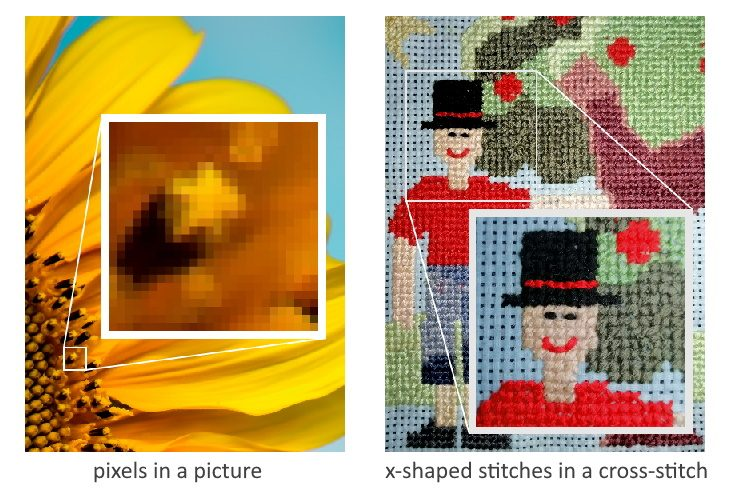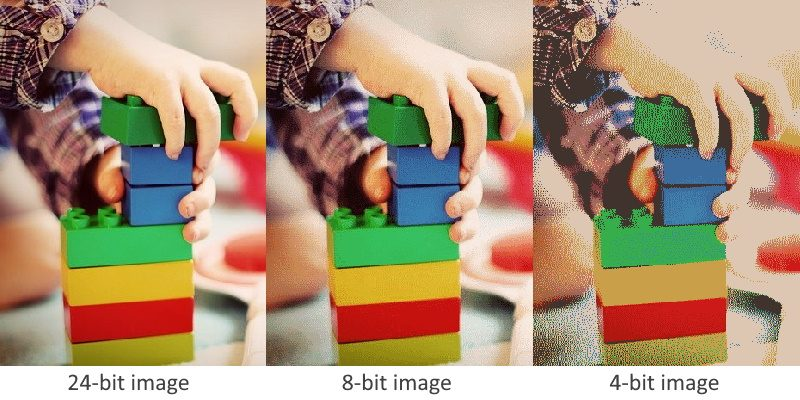# Image File Size Calculator

Created by Kenneth Alambra
Reviewed by Dominik Czernia, PhD candidate and Jack Bowater
Last updated: Apr 06, 2022

This image file size calculator will help you estimate the file size of an uncompressed raster image file, provided that you know the image's resolution and its bit depth.

In this calculator, you'll learn what an image file is, what bit depth means, and the difference between a raster image and a vector image. We'll also show you how to calculate image file sizes yourself and how combining it with an audio file creates a video file. Keep on reading to learn more.

## What is an image file?

An image file is a digital representation of a picture and we can display it on a screen like a computer monitor or a cell phone's screen. Image files contain data about a picture's attributes, like the colors present in it, brightness and contrast intensities, and much more. This data are stored in what we call bits. You can learn more about bits and computer files in the of our download time calculator.

Image files can come in many different file formats that we can group into two categories: raster and vector images. A raster image file contains an array of pixels of various colors arranged in a grid to form a picture. Pixels are tiny dots that are pretty much similar to the small X stitches in a cross-stitch pattern, as shown in the comparison picture below.Pictures with more pixels tend to show more details and more quality, especially when trying to zoom in on that image. A raster image with high pixel count, or resolution, lets us zoom in on the picture up a significant amount before we start to see the individual pixels that comprise it.

On the other hand, vector images consist of attributes like curves, paths, shapes, line or stroke thicknesses, colors, etc., that are stored in formulas or equations. Unlike raster images that store data for each pixel in an image, vector images store data as a compilation of these equations representing each of the said attributes. You can think of it as a list of mathematical equations that we can graph on a cartesian plane. Displaying a vector image is like graphing these equations on a cartesian plane every time we open the image file. These "graphs" also refresh every time we scale or zoom a vector image making us always see smooth lines and curves with consistent colors and details.

This image file size calculator, however, can only determine file sizes of raster images. Vector image attributes can vary an incredible amount, just like how you can express a mathematical graph with a variety of different mathematical equations. It's also worth noting that this image file size calculator only determines the file sizes of uncompressed images. However, you can even approximate a compressed image file size using this calculator if you know the image file's resulting bit depth.

## Understanding image file sizes

Two parameters define a raster image's file size, and these are the number of pixels in the image and the bit depth of each pixel. To determine the file size of a raster image file, we have to multiply these variables together. Each pixel in a raster image typically takes up about 1 to 8 bits for black-and-white images and 8 to 64 bits for colored images. And the more pixels an image has, the more data it stores, and the larger its file size.

We can determine the amount of bits per pixel with what we call "bit depth." What bit depth indicates is the number of colors that we can show in the image file. The number of colors in a certain bit depth follows the binary system and is equal to 2 raised to the bit depth value. For example, a 1-bit image can only show black or white pixels as 1 bit can only store two values, a 1 or a 0 (21 = 2 values or colors). On the other hand, a 16-bit image can use a total of 216 or 65,536 colors to represent a picture. We can then say that the higher the image file's bit depth is, the better the quality of the image will be. To visualize this, let us consider a picture that has been (or converted) to an image file with 24-bit, 8-bit, and 4-bit bit depths respectively:Sampling is obtaining information about a picture and trying to closely represent it in digital form in terms of pixels. We can also do it with sounds, wherein we record a variety of frequency and amplitude values to create a digital audio file. You can learn more about sampling through our audio file size calculator (see the link at the beginning of this article).

As you can see, the image that used a 24-bit bit depth is much more detailed than the 8-bit and 4-bit images. The 8-bit image starts to show imperfections in the least focused and least bright areas (also known as "noise"). These areas also become much more noisy and grainy in the 4-bit image.

## How to calculate image file size?

Now that we know what bit depth is and how pixels matter in determining what an image file's size is, let us consider an example to understand how to calculate image file sizes. For our example, let us take an image with the dimensions of, say, 640 pixels (width) by 480 pixels (height) at 24-bit bit depth. To determine the pixel count of this image, we multiply the dimensions of the image in pixels. It's just like obtaining the area of a rectangle but this time with pixels:

pixel count = width of image in pixels * height of image in pixels

pixel count = 640 pixels * 480 pixels

pixel count = 307,200 pixels

When talking about large numbers of pixels or resolutions, we can often see "MP," which stands for "megapixels." A megapixel is a unit equal to one million pixels. As for our calculated pixel count, we can also say that the image we are considering is approximately a 0.3-megapixel image. Continuing with our calculations, we can then determine the file size of our image as follows:

image file size = pixel count * bit depth

image file size = 307,200 pixels * 24 bits/pixel

image file size = 7,372,800 bits

image file size = 7,372,800 bits * (1 byte / 8 bits) * (1 kilobyte / 1000 bytes)

image file size = 921.6 KB (kilobytes)

Please note that our calculated image file size is just an estimate of the actual file size of the image file described. Computer files tend to have other data attached to them, including, but not limited to, the date the file was created, the name of the creator, and so on. But, for now, as an estimate of the image file size, we can say that it is around 921.6 KB. In our example above, we also used the SI decimal system to convert the data size, wherein 1000 byte is equivalent to 1 kilobyte. You can learn more about it in our download time calculator.

If you want to explore file sizes and the units used in digital files, you can check out our byte converter. There, you can input file sizes and see its value in other file size units - all at the same time.

Kenneth Alambra
Bit depth
24-bit (RGB true color)
Image width in pixels
pixels
Image height in pixels
pixels
Resolution
pixels
File size
MB
People also viewed…

### Aquarium glass thickness

The aquarium glass thickness calculator finds required aquarium thickness, volume, glass surface area, and weight for any given measurements.

### Circumference

Use this free circumference calculator to find the area, circumference and diameter of a circle.

### Grams to cups

The grams to cups calculator converts between cups and grams. You can choose between 20 different popular kitchen ingredients or directly type in the product density.

### PCB Impedance

Why would anyone calculate PCB impedances by hand, when creating some new electronics? Exactly! At Omni Calculator thought the same, and hence we have developed this PCB Impedance calculator: the only tool you'll need to get your numbers in a second.